## Aerodynamic Characteristics of Propellers

A propeller creates a thrust force out of the supplied power. The magnitude of this force is not constant for a given propeller, but depends on the velocity of the incoming air and the rotational velocity of the propeller itself. Thus tests of propellers usually cover a wide regime of operating conditions.

Propellers having the same shape, but scaled by a size factor behave similar. In order to make a comparison of propellers of different size easier, aerodynamicists try to get rid of the units. Then it is possible to use the results of a small scale wind tunnel model to predict the performance of a full scale propeller. Similar to airfoils and wings, the performance of propellers can be described by dimensionless (normalized) coefficients. While an airfoil can be characterized by relations between angle of attack, lift coefficient and drag coefficient, a propeller can be described in terms of advance ratio, thrust coefficient, and power coefficient. The efficiency, which corresponds to the L/D ratio of a wing, can be calculated from these three coefficients. These coefficients are helpful for the comparison of propellers of differing diameters, tested under different operating conditions.

Depending on the country where you live, there may be different coefficients in use. All coefficients used here, are based on the publications of the NACA, which defined the following coefficients:

 Thrust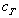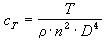Power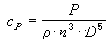Advance Ratio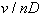Efficiency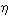where
 v velocity m/s D diameter m n revolutions per second 1/sdensity of air kg/mł P power W T thrust N

It should be noted, that the definition of the efficiency includes the velocity v. Thus the efficiency goes to zero when the flight speed approaches zero - of course, this does not mean, that the thrust goes to zero (see also: static thrust of propellers). Usually the power and thrust coefficients are plotted versus the advance ratio. The efficiency of a pylon racing model propeller, as calculated from these coefficients, is show below.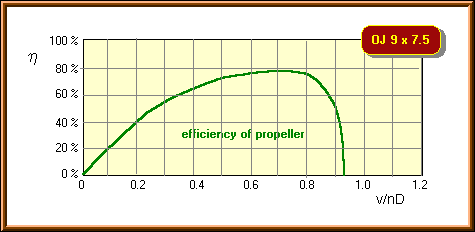A typical plot of efficiency versus advance ratio v/nD of a propeller for model airplanes.

Where do you want to go now?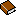Propulsion by Propellers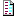Design a Prop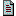Optimum PropellersDue to the increasing amount of SPAM mail, I have to change this e-Mail address regularly. You will always find the latest version in the footer of all my pages.

It might take some time until you receive an answer and in some cases you may even receive no answer at all. I apologize for this, but my spare time is limited. If you have not lost patience, you might want to send me a copy of your e-mail after a month or so.
This is a privately owned, non-profit page of purely educational purpose. Any statements may be incorrect and unsuitable for practical usage. I cannot take any responsibility for actions you perform based on data, assumptions, calculations etc. taken from this web page.